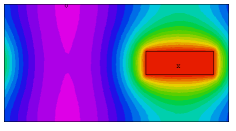﻿ Sample Problems > Usage > Periodicity > 3d_xperiodic

3d_xperiodic

Navigation:  Sample Problems > Usage > Periodicity >

3d_xperiodic{  3D_XPERIODIC.PDE

This example shows the use of FlexPDE in 3D applications with periodic boundaries.

The PERIODIC statement appears in the position of a boundary condition, but

the syntax is slightly different, and the requirements and implications are

more extensive.

The syntax is:

PERIODIC(X_mapping,Y_mapping)

The mapping expressions specify the arithmetic required to convert a point

(X,Y) in the immediate boundary to a point (X',Y') on a remote boundary.

The mapping expressions must result in each point on the immediate boundary

mapping to a point on the remote boundary.  Segment endpoints must map to

segment endpoints.  The transformation must be invertible; do not specify

constants as mapped coordinates, as this will create a singular transformation.

The periodic boundary statement terminates any boundary conditions in effect,

and instead imposes equality of all variables on the two boundaries.  It is

still possible to state a boundary condition on the remote boundary,

but in most cases this would be inappropriate.

The periodic statement affects only the next following LINE or ARC path.

These paths may contain more than one segment, but the next appearing

LINE or ARC statement terminates the periodic condition unless the periodic

statement is repeated.

In this problem, we have a heat equation with an off=center source in an irregular

figure.  The figure is periodic in X, with Y faces held at zero, and Z-faces insulated.

 }   title '3D X-PERIODIC BOUNDARY TEST'   coordinates    cartesian3   Variables    u   definitions    k = 0.1    h=0    x0=0.5  y0=-0.2    x1=1.1  y1 = 0.2   equations    u : div(K*grad(u)) + h = 0   extrusion z=0,0.4,0.6,1   boundaries   region 1     start(-1,-1)value(u)=0   line to (1,-1)   { Force U=0 on Y=-1 }

{ The following arc is required to be a periodic image of an arc

two units to its left. (This image boundary has not yet been defined.) }

periodic(x-2,y) arc(center=-1,0) to (1,1)

value(u)=0   line to (-1,1)       { Force U=0 on Y=1 }

{ The following arc provides the required image boundary for the previous

periodic statement }

nobc(u)   { turn off the value BC }

arc(center= -3,0) to close

{ an off-center heat source in layer 2 provides the asymmetric conditions to

demonstrate the periodicity of the solution }

limited region 2

layer 2 h=10 k=10

start(x0,y0) line to (x1,y0) to (x1,y1) to (x0,y1) to close

monitors

contour(u) on z=0

contour(u) on z=0.5

contour(u) on z=1

contour(u) on y=0

plots

contour(u) on z=0   painted

contour(u) on z=0.5 painted

contour(u) on z=1   painted

contour(u) on y=0   painted

end